# Density Modelling in Mixed Convection Flow in a Vertical Parallel Plate Channel

Density Modelling in Mixed Convection Flow in a Vertical Parallel Plate Channel
NTPC, a Power Sector Company, an Indian Public Sector Undertaking, New Delhi 110003, India

Corresponding Author Email:
przsiddiqui@gmail.com
Page:
1294-1304
|
DOI:
https://doi.org/10.18280/ijht.390428
29 January 2020
|
Accepted:
27 July 2021
|
Published:
31 August 2021
| Citation

OPEN ACCESS

Abstract:

In this paper, a novel way of modelling the density in buoyancy term of mixed convection flow problem is presented using equation of state and Boussinesq approximation without first-order approximation of density with respect to temperature. The presented density model is used to investigate the laminar mixed convection flow in a vertical parallel plate channel under symmetric constant wall heat flux. The results obtained are compared with the results obtained using first-order approximation of density with Boussinesq approximation, and also compared with the results obtained using variable thermophysical properties with negligible viscous dissipation. Investigation is performed on the basis of flow and thermal fields for Re=150 and 300, Ri=0.1 to 25. It is found that the presented density model produces relatively better results, which is able to describe the case of developing flow under constant heat flux condition that is not evident if Boussinesq approximation with first-order approximation of density is used. An appearance of recirculatory cells when reverse flow takes place is also witnessed in vertical channel flow with constant heat flux boundary condition which was not reported earlier.

Keywords:

mixed convection, buoyancy, density, Boussinesq approximation, reverse flow, vertical channel

1. Introduction

Mixed convection heat transfer in a vertical channel has many applications in equipment such as heat exchanger, solar flat plate collector, electronic chips, nuclear reactor and so on. Previously, mixed convection with constant temperature or constant heat flux boundary conditions in a parallel plate channel has been solved analytically, numerically, and experimentally. The main task involves in solving the mixed convection problem is handling the thermophysical properties specially density appeared in conservation equation of mass, momentum and energy transfer. Various works have been performed earlier to address the variation of thermophysical properties with respect to temperature and pressure. One of the breakthroughs in this regard is well known Boussinesq approximation.

Some of the previous benchmark works using Boussinesq approximation are mentioned subsequently. Aung and Worku  numerically solved the mixed convection flow in a parallel plate duct with uniform wall heat flux for Gr/Re varied from 0 to 500. They have observed dynamically developed flow for symmetrically heated wall when the duct length is long enough. Aung and Worku  studied the developing flow and flow reversal in a vertical parallel plate channel with asymmetric wall temperatures. They have numerically solved the boundary layer equations and observed the effects of buoyancy on the hydrodynamic and thermal parameters. Habchi and Acharya  solved the laminar mixed convection in a symmetrically and asymmetrically heated vertical channel and explained the physics of the flow for Gr/Re2 varied from 0.1 to 5. Ingham et al.  numerically solved the problem of steady laminar mixed convection flow in a vertical parallel plate channel with symmetric wall temperatures for Gr/Re ratio varied from -300 to 70. They observed the occurrence of flow reversal for a large value of Gr/Re ratio. Jeng et al.  numerically solved and examined the mixed convection in a vertical channel subjected to asymmetric wall temperature with and without flow reversal over the range of Re from 1 to 1000 and Gr/Re ratio varied from 0 to 500. Cheng et al.  studied the buoyancy-assisted flow reversal and convective heat transfer in a vertical rectangular duct for various heating conditions. Moukalled et al.  numerically solved and studied mixed convection in channels with concave and convex surfaces for various curvature ratio of concave and convex wall. Balaji and Premachandran  studied mixed convection heat transfer flow inside converging, parallel and diverging channel with uniform volumetric heat generating plates for a wide range of Re, Gr, thermal conductivity ratio and angle of convergence or divergence. Desrayaud and Lauriat  solved the mixed convection heat transfer flow inside a vertical parallel plate channel with symmetric constant wall temperature. They studied the flow reversal phenomena at the entry region of channel. Dritselis et al.  studied buoyancy-assisted mixed convection in a vertical channel with spatially periodic wall temperature. They have observed multi-cellular regime of flow when Gr/Re value is above a critical ratio. Zghal et al.  studied the developing mixed convection flow in vertical tube with constant heat flux on the wall. They investigated the effect of heating length on flow fields and reverse flow. They observed that for a longer heating length, a fully developed condition is achieved.

All the literature mentioned above have investigated the mixed convection flow with constant wall temperature or constant wall heat flux using Boussinesq approximation with first-order approximation of density in buoyancy term. Also, to simplify the computation, they have assumed constant thermophysical properties. These thermophysical properties are generally evaluated at film temperature for the flow when there is constant temperature boundary condition. However, in case of heat flux boundary condition, film temperature cannot be correctly determined in advance, therefore thermophysical properties in this case are evaluated at inlet condition. With this approximation, physics of flow and trend of the derived parameters have been explained but this is not sufficient to produce the actual condition of flow field in case of wall heat flux boundary condition, whether the flow field is developed or still developing, recirculatory cells are appeared or not during reverse flow.

It is observed that with boundary condition of wall heat flux, flow is not developed in reality because of change in density of the fluid as the flow proceeds, which has been observed in previous works using thermophysical properties as a function of temperature. Chato and Lawrence  did numerical and experimental study of laminar mixed convection flow inside vertical tubes. They have assumed density as a non-linear function of temperature in buoyancy term, elsewhere it is taken as a constant. They have also assumed viscosity as a non-linear function of temperature. With this assumption they observed that velocity and temperature profiles constantly change and never reach fully developed states for constant heat flux condition. Gray and Giorgini  recommended linearized approximation of thermophysical properties with respect to temperature and pressure in conservation equations. They have obtained the conditions under which Boussinesq approximation is valid. Fu et al.  solved the natural convection flow in an asymmetrically heated wall without Boussinesq approximation. They have used density and viscosity as a function of primitive variables in conservation equations. Talukdar et al.  studied compressible laminar natural-convection for a staggered and symmetric arrangement of discrete heat sources in an open-ended vertical channel using non-Boussinesq approximation by considering density and viscosity as a function of temperature. Grelf  also did an experimental and theoretical study of heat transfer in vertical tube flows with constant heat fluxes. They found that the velocity and temperature profiles never become “fully developed” and attributed this to the non-linear variation of density and viscosity with temperature. Recently, various authors have studied the effect of density variation using non-linear Boussinesq approximation. Jah and Oni  studied the effect of non-linear Boussinesq approximation on mixed convection flow in a vertical channel. They have adopted non-linear density variation with temperature in the buoyancy term, which resulted in increased fluid velocity, skin friction coefficient and increased reverse flow formation. Rajeev and Mahanthesh  studied the multilayer flow and heat transport of nano-liquids with non-linear Boussinesq approximation to simulate the effect of density variation.

Correct evaluation of thermophysical properties specially density in buoyancy term of mixed convection flow problem is very important to get the accurate results. It is observed from literature review using Boussinesq approximation with first-order approximation of density (called as model 1), hydrodynamically developed condition is achieved in mixed convection flow in a vertical channel with symmetrical heat flux, which does not happen in reality and this has been explained in the work of Chato and Lawrence . Also, the presence of reverse flow and recirculatory cells is very much governed by the variation of density appearing in buoyancy term. Therefore, the density modelling plays an important role in getting the accurate results.

For the fluid which has equation of state, density in buoyancy term may be approximated without first-order approximation. In this study, density appearing in buoyancy term is modelled using ideal gas equation without first-order approximation of density (called as model 2). The solutions obtained using model 1 and 2 are compared with the solution of full-fledged governing equations with variable thermophysical properties and negligible viscous dissipation (called as model 3) based on flow and thermal field for a range of governing parameters. Regime of reverse flow and recirculatory cells are also discussed using models 1, 2 and 3.

2. Problem Definition and Mathematical Formulation

The mathematical domain consists of a vertical parallel plate channel of width, w and height, h. Fluid at ambient temperature, T∞ and uniform velocity, v∞ enters at bottom of the channel as shown in Figure 1. Mixed convection flow inside the channel is modelled as: (a) 2-D laminar, incompressible flow with negligible viscous dissipation and constant thermophysical properties in which the Boussinesq approximation is considered to be valid, and the density terms are modelled as per model 1 and model 2 (explained in section 2.2) (b) 2-D laminar flow with negligible viscous dissipation and variable thermophysical properties, where the density terms are modelled as per model 3 (explained in section 2.2). Symmetric constant heat flux is imposed over left and right wall of the vertical channel. Fluid considered is air, which obeys the ideal gas equation of state.

## 1.png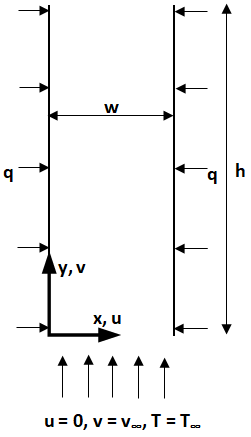Figure 1. Schematic of the flow in a parallel plate vertical channel

2.1 Governing equations

The governing mass, momentum and energy conservation equations for 2-D laminar, incompressible mixed convection flow with negligible viscous dissipation in dimensionless form are:

$\frac{\partial U}{\partial X}+\frac{\partial V}{\partial Y}=0$    (1a)

$U \frac{\partial U}{\partial X}+V \frac{\partial U}{\partial Y}=-\frac{\partial P}{\partial X}+\frac{1}{R e}\left(\frac{\partial^{2} U}{\partial X^{2}}+\frac{\partial^{2} U}{\partial Y^{2}}\right)$   (1b)

$U \frac{\partial V}{\partial X}+V \frac{\partial V}{\partial Y}=-\frac{\partial P}{\partial Y}+\frac{1}{R e}\left(\frac{\partial^{2} V}{\partial X^{2}}+\frac{\partial^{2} V}{\partial Y^{2}}\right)+B$    (1c)

$U \frac{\partial \theta}{\partial X}+V \frac{\partial \theta}{\partial Y}=\frac{1}{\operatorname{RePr}}\left(\frac{\partial^{2} \theta}{\partial X^{2}}+\frac{\partial^{2} \theta}{\partial Y^{2}}\right)$    (1d)

The full-fledged governing mass, momentum and energy conservation equations for 2-D laminar mixed convection flow with variable thermophysical properties and negligible viscous dissipation in dimensionless form are:

$\frac{\partial \rho^{*} U}{\partial X}+\frac{\partial \rho^{*} V}{\partial Y}=0$   (2a)

\begin{aligned} U \frac{\partial \rho^{*} U}{\partial X}+V \frac{\partial \rho^{*} U}{\partial Y} \\ &=-\frac{\partial P}{\partial X} \\ &+\frac{1}{R e}\left\{2 \frac{\partial}{\partial X}\left(\mu^{*} \frac{\partial U}{\partial X}\right)\right.\\ &+\frac{\partial}{\partial Y}\left[\mu^{*}\left(\frac{\partial U}{\partial Y}+\frac{\partial V}{\partial X}\right)\right] \\ &\left.-\frac{\partial}{\partial X}\left[\frac{2}{3} \mu^{*}\left(\frac{\partial U}{\partial X}+\frac{\partial V}{\partial Y}\right)\right]\right\} \end{aligned}    (2b)

\begin{aligned} U \frac{\partial \rho^{*} V}{\partial X}+V \frac{\partial \rho^{*} V}{\partial Y} & \\ &=-\frac{\partial P}{\partial Y} \\ &+\frac{1}{R e}\left\{\frac{\partial}{\partial X}\left[\mu^{*}\left(\frac{\partial U}{\partial Y}+\frac{\partial V}{\partial X}\right)\right]\right.\\ &+2 \frac{\partial}{\partial Y}\left(\mu^{*} \frac{\partial V}{\partial Y}\right) \\ &\left.-\frac{\partial}{\partial X}\left[\frac{2}{3} \mu^{*}\left(\frac{\partial U}{\partial X}+\frac{\partial V}{\partial Y}\right)\right]\right\}+B \end{aligned}    (2c)

$\begin{array}{r} U \frac{\partial \rho^{*} c_{p}^{*} \theta}{\partial X}+V \frac{\partial \rho^{*} c_{p}^{*} \theta}{\partial Y} \\ =\frac{1}{\operatorname{RePr}}\left\{\frac{\partial}{\partial X}\left(k_{f}^{*} \frac{\partial \theta}{\partial X}\right)\right. \\ \left.+\frac{\partial}{\partial Y}\left(k_{f}^{*} \frac{\partial \theta}{\partial Y}\right)\right\} \end{array}$   (2d)

where, B is the buoyancy term which is defined in sec-2.2 and the dimensionless parameters are defined as follows:

$\begin{gathered} X=\frac{x}{w}, Y=\frac{y}{w}, U=\frac{u}{v_{\infty}}, V=\frac{v}{v_{\infty}}, P=\frac{p}{\rho_{\infty} v_{\infty}^{2}} \\ \Delta T_{\text {ref }}=\frac{q w}{k_{f}}, \\ \theta=\frac{T-T_{\infty}}{\Delta T_{r e f}}, \rho^{*}=\frac{\rho}{\rho_{\infty}}, \mu^{*}=\frac{\mu}{\mu_{\infty}}, c_{p}^{*}=\frac{c_{p}}{c_{p \infty}}, k_{f}^{*}= \\ \frac{k_{f}}{k_{f \infty}} \\ \begin{array}{c} R e=\frac{v_{\infty} w}{v}, \quad G r=\frac{\beta g \Delta T_{r e f} w^{3}}{v^{2}}, \quad R i=\frac{G r}{R e^{2}}, \\ & P r=\frac{v}{\alpha} \end{array} \end{gathered}$       (3)

Equation of state:

$p=\rho R T$      (4)

At wall, velocity components are obtained from no-slip condition and temperature is obtained from constant heat flux condition imposed at wall. Fluid is assumed to enter the channel at uniform axial velocity, zero transverse velocity and at ambient temperature. At exit of the channel, second derivatives of U, V and θ are taken as zero for smoothing out the variations in U, V and θ . Boundary conditions are as follows:

Left wall: X = 0, 0 < Y < A, U = 0, V = 0,  $\frac{\partial \theta}{\partial X}=-1$   (5)

Right wall: X = 1, 0 < Y < A, U = 0, V = 0,  $\frac{\partial \theta}{\partial x}=1$    (6)

Inlet: 0 < X < 1, Y = 0, U = 0, V = 1, θ = 0    (7)

Exit:  0 < X < 1, Y = A,  $\frac{\partial^{2} U}{\partial Y^{2}}=0, \frac{\partial^{2} V}{\partial Y^{2}}=0, \frac{\partial^{2} \theta}{\partial Y^{2}}=0$    (8)

2.2 Density modelling

Model 1:

Density appearing in numerator of convection terms is assumed as constant, and it is shifted to denominator of diffusion and buoyancy terms. The density appeared in denominator of diffusion terms and buoyancy term are determined at inlet temperature. The buoyancy term is modelled using first-order approximation of density with respect to temperature and volumetric expansion coefficient, β is incorporated in buoyancy term. With this manipulation, a set of equations 1a-d is obtained. All the involved thermophysical properties are evaluated at the inlet temperature. Buoyancy term is modelled as:

B = Riθ    (9)

Model 2:

All the thermophysical properties are evaluated in the same manner as in model 1. Here, the buoyancy term is modelled using ideal gas equation without first-order approximation, which is as follows:

$\begin{gathered} B=\frac{\left(\rho_{\infty}-\rho\right)}{\rho_{\infty}} \frac{g w}{V_{\infty}^{2}}=\left(1-\frac{\rho}{\rho_{\infty}}\right) \frac{g w}{V_{\infty}^{2}}=\left(1-\frac{p}{p_{\infty}} \frac{T_{\infty}}{T}\right) \frac{g w}{V_{\infty}^{2}} \\ =\left(1-p^{*}\left(\frac{T_{\infty}-T}{T}+1\right)\right) \frac{g w}{V_{\infty}^{2}} \\ B=\left(1-p^{*}+\frac{p^{*}}{T^{*}} \frac{\theta \Delta T_{r e f}}{T_{\infty}}\right) \frac{g w}{V_{\infty}^{2}} \\ B=\left(1-p^{*}\right) \frac{g w}{V_{\infty}^{2}}+\frac{p^{*}}{T^{*}} \frac{\theta \Delta T_{\text {ref }}}{T_{\infty}} \frac{g w}{V_{\infty}^{2}} \\ B=\frac{\left(1-p^{*}\right)}{F r^{2}}+\frac{p^{*}}{T^{*}} \frac{\beta_{\infty} g \Delta T_{r e f} w^{3}}{v^{2}} \frac{1}{R e^{2}} \theta \\ B=\frac{\left(1-p^{*}\right)}{F r^{2}}+\rho^{*} \operatorname{Ri} \theta \end{gathered}$

$B=\frac{\left(1-p^{*}\right)}{F r^{2}}+\rho^{*} \operatorname{Ri\theta}$       (10)

where, $F r=\frac{V_{\infty}}{(g w)^{\frac{1}{2}}}, p^{*}=\frac{p}{p_{\infty}} T^{*}=\frac{T}{T_{\infty}}$.

p* may be approximated to unity since the change in absolute pressure is not significant for the present computational domain. Therefore, the buoyancy term may be approximated as:

$B=\frac{1}{T^{*}} \operatorname{Ri} \theta$    (11)

Model 3:

All the thermophysical properties are considered as a function of temperature. Formulation of buoyancy term, B is same as described in model 2.

As per author’s knowledge, model 2 has not been used previously to solve the laminar mixed convection problem. In this paper, model 2 is numerically solved and evaluated by comparing with the numerical solution obtained using model 1 and 3 for the domain as mentioned above.

2.3 Nusselt number and friction factor

The local and average Nusselt numbers based on fluid bulk temperature are defined as follows:

\begin{aligned} N u_{y}=\left.\frac{2}{1-\theta_{b}} \frac{\partial \theta}{\partial X}\right|_{\text {at wall }}, \theta_{b} \\ =& \frac{\int_{0}^{1} \rho^{*} c_{p}^{*} V \theta d X}{\int_{0}^{1} \rho^{*} c_{p}^{*} V d X}, N u_{a v g} \\ =\frac{1}{A} \int_{0}^{A} N u_{y} d Y \end{aligned}      (12)

The local and average Nusselt numbers based on ambient temperature are defined as follows:

$N u_{y, \infty}=\left.2 \frac{\partial \theta}{\partial X}\right|_{\text {at wall }}, \quad N u_{\text {avg, } \infty}=\frac{1}{A} \int_{0}^{A} N u_{y} d Y$      (13)

The local and average coefficients of friction are defined as follows:

$C_{f y}=\left.\frac{4}{R e} \mu^{*} \frac{\partial V}{\partial X}\right|_{\text {at wall }}, \quad C_{\text {favg }}=\frac{1}{A} \int_{0}^{A} C_{f Y} d Y$      (14)

2.4 Correlation for thermophysical properties

Dynamic viscosity in kg/m. s for air is evaluated from Sutherland law:

$\begin{gathered} \mu(T)=\mu_{0}\left(\frac{T}{T_{0}}\right)^{1.5}\left(\frac{T_{0}+S}{T+S}\right) \\ S=110.4 K, T_{0}=273 K, \\ \mu_{0}=1.71 * 10^{-5} \end{gathered}$    (15)

Correlation for thermal conductivity in W/m. K and specific heat capacity in J/kg. K for air are as follows:

\begin{aligned} k_{f}(T)=1.5207 * & 10^{-11} T^{3}-4.8574 * 10^{-8} T^{2} \\ &+1.0184 * 10^{-4} T-3.9333 \\ & * 10^{-4} \end{aligned}      (16)

\begin{aligned} c_{p}(T)=34.518 * &\left(28.11+0.1967 * 10^{-2} T\right.\\ &+0.4802 * 10^{-5} T^{2}-1.966 \\ &\left.* 10^{-9} T^{3}\right) \end{aligned}       (17)

where, T is absolute temperature in Kelvins.

3. Numerical Method and Details

Finite volume method [19, 20] is used to discretize a set of dimensionless governing elliptic differential equations on non-uniform staggered grid. Upwind differencing scheme for convective terms, and central differencing scheme for diffusive terms are used for discretization. Algorithm used for solving the conservation equations is a modified form of SIMPLE algorithm known as Coupled and Linked Equations Algorithm Revised (CLEAR), a fully implicit method, which shows improved robustness and faster convergence amongst family of SIMPLE algorithm . The derived algebraic equations are solved by tri-diagonal matrix algorithm (TDMA) over the computational domain. Cartesian non-uniform grids are generated over the computational domain which is finer near wall and at inlet, and coarser elsewhere using Cosine and Semi Cosine functions in X and Y directions respectively. An in-house computer code is written for implementation of the algorithm. The iteration is carried out by assuming arbitrary values for all the dependent variables in the flow domain. A suitable under-relaxation factor for U and V components are used for getting the converged solution. The solution is accepted for convergence when the relative variation in dependent variables U, V and θ become less than 10-5.

4. Results and Discussion

Laminar mixed convection flow in a 2-D symmetrically heated vertical parallel plate channel is solved for Re = 150 and 300, and Ri = 0.1 to 25 using models 1, 2 and 3. Channel aspect ratio is fixed at A = 50, and the ambient temperature is fixed at 20 °C. A uniform heat flux of q = 100 W/m2 is imposed on both the left and right walls.

As the flow proceeds inside the channel, θ increases and ρ* decreases, which modulates the buoyancy term. However, the buoyancy term, B in model 1 is modulated by variable dimensionless temperature, θ and a constant Ri, while the buoyancy term in model 2 is modulated by variable dimensionless temperature, θ, variable density ratio, ρ* and a constant Ri. Therefore, the formulation of buoyancy term in model 2 closely follows the physical condition inside the channel. Since model 3 assumes variable thermophysical properties with only assumption of negligible viscous dissipation, therefore, the results obtained using model 3 is considered as a reference for comparative evaluation of model 1 and model 2.

4.1 Grid independency test

In order to ensure the grid independency of results, models 1, 2 and 3 are solved for 2-D laminar mixed convection flow in a vertical channel with symmetrical constant heat flux of q=100W/m2 for Re=150, Ri=1, A=50. Average Nusselt number in the channel, Nuavg, average coefficient of friction at the channel wall, Cfavg, centreline maximum axial velocity in the channel, Vmax, centreline maximum temperature in the channel, θmax at centre, and maximum wall temperature at the channel wall, θwmax are computed using model 1 (Table 1), model 2 (Table 2) and model 3 (Table 3) on successive grid sizes. The grid size is mentioned as MxN, where M and N show number of grid points in x and y directions respectively. It is observed from Table 1, 2 and 3 as the grid refines from 31×151 to 61×301, percentage change in the derived parameters decreases and improvement in the value of derived parameters decreases, therefore, a grid size of 51×251 can be selected for a satisfactory solution.

4.2 Validation

The present numerical method is validated with both the numerical and experimental work. Desrayaud  has numerically solved the 2-D laminar mixed convection flow under symmetrical constant wall temperature using Boussinesq approximation and first-order approximation of density, which is based on the model 1 as mentioned in section 2.2. Therefore, the presented model 1 is validated with Desrayaud work for 2-D laminar mixed convection flow in a vertical channel under symmetric constant wall temperature. It is observed that centreline axial velocity (Figure 2a), bulk and centreline temperature (Figure 2b) and local bulk Nusselt number (Figure 2c) obtained using model 1 are in close agreement with the results obtained in the work of Desrayaud . The results obtained from other model 2 and 3 are presented and discussed along with the results of model 1 in the subsequent sections.

Table 1. Grid independency test for successive grids using model 1, Re = 150, Ri = 1, A = 50

 M N Average Nusselt number (Nuavg) Absl change (%) Average coeff. of friction (Cfavg) Absl change (%) Vmaxat centreline of channel Absl change (%) θmax at centreline of channel Absl change (%) θmax at wall of channel Absl change (%) 31 151 9.5275 0.2737 1.3270 0.8780 1.1747 41 201 9.5365 0.0949 0.2741 0.1220 1.3274 0.0344 0.8774 0.0714 1.1740 0.0674 51 251 9.5419 0.0560 0.2744 0.1285 1.3277 0.0206 0.8769 0.0519 1.1734 0.0446 61 301 9.5455 0.0381 0.2748 0.1188 1.3279 0.0141 0.8766 0.0384 1.1731 0.0314

Table 2. Grid independency test for successive grids using model 2, Re = 150, Ri = 1, A = 50

 M N Average Nusselt number (Nuavg) Absl change (%) Average coeff. of friction (Cfavg) Absl change (%) Vmaxat centreline of channel Absl change (%) θmax at centreline of channel Absl change (%) θmax at wall of channel Absl change (%) 31 151 9.4246 0.2598 1.3558 0.8786 0.9506 41 201 9.4339 0.0989 0.2602 0.1490 1.3555 0.0239 0.8780 0.0769 0.9498 0.0824 51 251 9.4394 0.0586 0.2606 0.1470 1.3553 0.0134 0.8775 0.0522 0.9493 0.0523 61 301 9.4432 0.0406 0.2609 0.1334 1.3552 0.0101 0.8772 0.0345 0.9490 0.0316

Table 3. Grid independency test for successive grids using model 3, Re = 150, Ri = 1, A = 50

 M N Average Nusselt number (Nuavg) Absl change (%) Average coeff. of friction (Cfavg) Absl change (%) Vmaxat centreline of channel Absl change (%) θmax at centreline of channel Absl change (%) θmax at wall of channel Absl change (%) 31 151 9.1277 0.2737 1.6674 0.9825 1.0561 41 201 9.1377 0.1095 0.2740 0.1420 1.6664 0.0600 0.9817 0.0765 1.0554 0.0701 51 251 9.1431 0.0595 0.2744 0.1284 1.6659 0.0284 0.9808 0.0910 1.0543 0.0985 61 301 9.1476 0.0490 0.2748 0.1382 1.6654 0.0267 0.9809 0.0105 1.0547 0.0325

The benchmark experimental work by Elenbaas  about free convection heat dissipation in parallel plates is taken for further validation. For validation with the Elenbaas work, in the present work, Ri value is fixed at 100, which is quite sufficient for establishing free convection dominant flow in a vertical parallel plate channel. Total twenty values of Nuavg,∞ (based on ambient temperature) are obtained at Ri = 100 for aspect ratios A=4 to 50 using model 1 for 2-D laminar mixed convection flow in a parallel plate vertical channel under symmetric constant temperature condition on walls. The present numerical results and the results obtained from semi-experimental correlation available in Elenbaas work  are plotted in Pareto chart (Figure 3) where y axis corresponds to Nusselt number of the present work and x axis corresponds to Nusselt number of Elenbaas work. It is observed from Pareto chart (Figure 3) that the obtained results are found in agreement with the Elenbaas work with the maximum deviation $\left(100 * \frac{N u_{\text {reference }-N u_{\text {present work }}}}{N u_{\text {reference }}} \%\quad\quad\right)$  of 9.1%.

## 2-1.png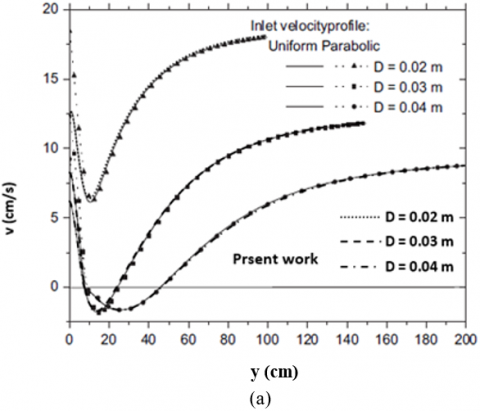Figure 2. Validation with the work of Desrayaud and Lauriat  (a) centreline axial velocity profile, (b) bulk and centreline temperature, (c) local bulk Nusselt number

## 2-2.png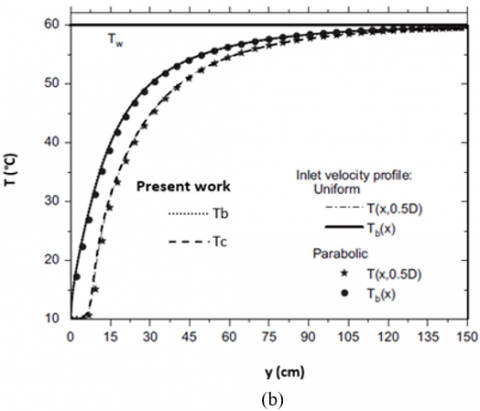## 2-3.png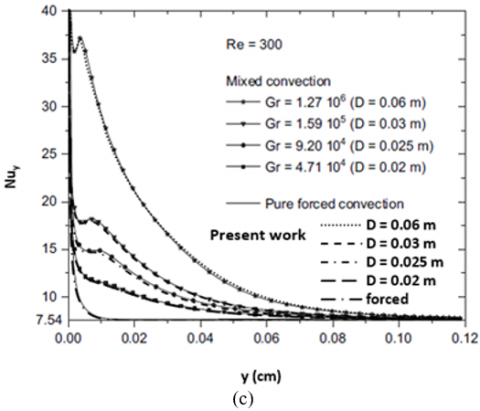Figure 3. Pareto chart for Comparison of Nuavg,∞ between the present work and work of Elenbaas  for A = 4 to 50

4.3 Velocity profiles along the channel width

Figure 4a, b shows the axial velocity profile at Y=6, Re=150 for Ri=0.1 and 10 respectively using models 1, 2 and 3. It is observed that at Ri=0.1, the axial velocity profiles are almost the same because of slight difference in thermophysical properties amongst models 1, 2 and 3 near channel inlet. However, as Ri increases, slight difference in velocity profile appears amongst models 1, 2 and 3. At Ri=10, axial velocity near wall increases because of buoyancy and decreases at centerline for maintaining the conservation of mass. In center region, model 3 predicts slightly higher axial velocity and model 1 predicts slight lower axial velocity, while model 2 predicts axial velocity which lies in between axial velocity of model 1 and 3.

Figure 5a, b shows the axial velocity profile at channel exit, Re=150 for Ri=0.1 and 10 respectively using models 1, 2 and 3. At Ri=0.1, model 1 and 2 predicts almost the same axial velocity profile, while model 3 predicts higher axial velocity because of lower density at higher temperature. However, all models 1, 2 and 3 predict the same axial velocity near wall, which is governed by buoyancy and no slip boundary condition at wall. At Ri=10, near wall model 1 predicts slightly higher axial velocity, while model 2 predicts slightly lower axial velocity and model 3 predicts axial velocity in between the axial velocity of model 1 and 2. In center region, model 1 predicts lower axial velocity and model 3 predicts higher axial velocity, while model 2 predicts axial velocity which lies in between axial velocity of model 1 and 3.

Overall axial velocity profile of model 2 as compared to model 1, is closer to the axial velocity profile of model 3, which is observed for Re=150, Ri=0.1 and 10 at both inlet (Figure 4) and exit (Figure 5) of the channel.

4.4 Temperature profiles along the channel width

Figure 6a, b shows the temperature distribution at Y=6, Re=150 for Ri=0.1 and 10 respectively using models 1, 2 and 3. It is observed that at Ri=0.1, the temperature profiles are almost the same because of slight difference in thermophysical properties amongst models 1, 2 and 3 near channel inlet. However, as Ri increases, slight difference in temperature profile appears amongst models 1, 2 and 3. At Ri=10, model 3 predicts slightly higher temperature, while model 1 and 2 predicts almost the same temperature.

Figure 7a, b shows the temperature distribution at channel exit, Re=150 for Ri=0.1 and 10 respectively using models 1, 2 and 3. It is observed that at Ri=0.1, model 1 and 2 predicts almost the same temperature, while model 3 predicts significantly high temperature. The reason for rise in temperature in model 3 is due to less increase in specific heat capacity as compared to large drop in density, which causes high temperature near wall due to almost same velocity near wall as in model 1 and 2. Also, the decrease in temperature from wall to centerline is higher in model 3 because of higher axial velocity at centerline, which is more evident as Ri increases from 0.1 to 10. As Ri increases, temperature profile of model 1 and 2 starts deviating from each other. At Ri=10, model 2 as compared to model 1 predicts higher temperature near wall and slight lower temperature in center region. Model 3 as compared to both model 1 and 2, always predicts significantly higher temperature as Ri increases.

Overall temperature profile of model 2 as compared to model 1, is closer to the temperature profile of model 3, which is more evident for Re = 150, Ri = 10 at exit (Figure 7) of the channel.

4.5 Velocity and temperature profiles along the channel centreline

Figure 8 shows the centerline axial velocity profile for Re=150, Ri=0.1, 1, 5, 10 and 25. Near inlet region, all models 1, 2 and 3 predicts almost the same centerline axial velocity for the studied value of Re and Ri. At Ri=0.1, model 1, 2 predicts almost the same axial velocity throughout the channel, while model 3 predicts axial velocity which is increasing in axial direction and it is higher than the axial velocity of model 1 and 2. At lower value of Ri, difference in trend of axial velocity between the model 1 and 2 are not much evident, however, as Ri increases, model 2 starts deviating from model 1 and approaches towards model 3. Model 1 approaches towards fully developed flow for all the values of Ri=0.1, 1, 5, 10 and 25, while model 2 and 3 are showing developing flow because of increasing centerline axial velocity in axial direction. At Ri=25, model 1 predicts centerline axial velocity which is dropping to negative value in the inlet region and then increases to reach a constant negative velocity, model 2 predicts centerline axial velocity which is also dropping to a negative value in the entrance region and then monotonically increases till the channel exit, and model 3 predicts the centerline axial velocity also in the same manner as model 2 but with increased value. Since, density of the fluid decreases as the flow proceeds, therefore, at Ri=25, centerline axial velocity of model 3 approached to less negative value.

## 4.png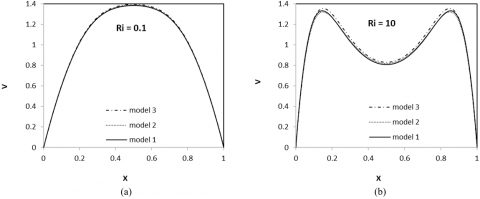Figure 4. Axial velocity (dimensionless) at Y=6 using models 1, 2 and 3, for A=50, Re=150, (a) Ri=0.1, (b) Ri=10

## 5.png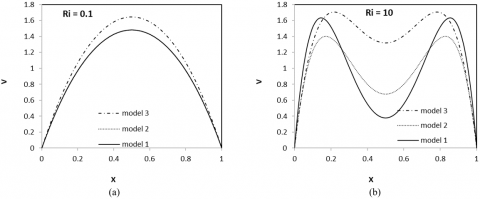Figure 5. Axial velocity (dimensionless) at channel exit using models 1, 2 and 3, for A=50, Re=150, (a) Ri=0.1, (b) Ri=10

## 6.png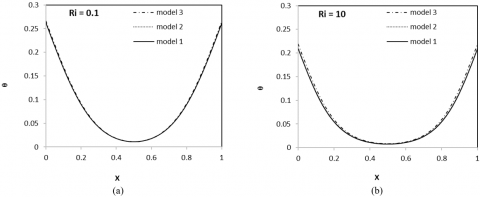Figure 6. Temperature (dimensionless) distribution at Y=6 using models 1, 2 and 3, for A=50, Re=150, (a) Ri=0.1, (b) Ri=10

## 7.png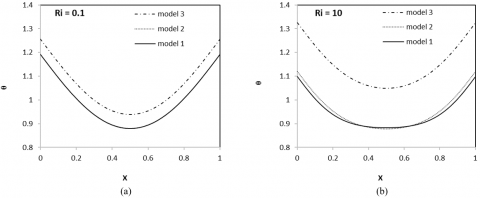Figure 7. Temperature (dimensionless) distribution at channel exit using models 1, 2 and 3, for A=50, Re=150, (a) Ri=0.1, (b) Ri=10

Centerline axial velocity profile of model 2 as compared to model 1, is always closer towards the centerline axial velocity profile of model 3, and shows developing flow. It is observed from the centerline axial velocity profile at Ri=25, where buoyancy force is dominant, model 2 and 3 predicts recirculatory cells in reversed flow region, while model 1 predict reverse flow and failed to predict recirculatory cells in reverse flow region which is observed in Figure 10.

Figure 9 shows the distribution of difference between wall temperature and bulk temperature (θw – θb) in axial direction for Re=150, Ri=0.1, 1, 5, 10 and 25. At Ri = 0.1, model 1, 2 predicts almost the same temperature difference (θw – θb) throughout the channel, while model 3 predicts temperature difference (θw – θb) which is increasing in axial direction and it is higher than the temperature difference (θw – θb) of model 1 and 2. At lower value of Ri, difference in trend of temperature difference (θw – θb) between the model 1 and 2 are not much evident, however, as Ri increases, temperature difference of model 2 starts deviating from temperature difference of model 1, and temperature difference of model 2 as compared to model 1, approaches towards the temperature difference of model 3. At higher values of Ri, temperature difference first increases and decreases near inlet region in all the models 1, 2 and 3, and then approaches towards constant value for model 1, while for model 2 and 3, temperature difference increases till the channel exit.

## 8.png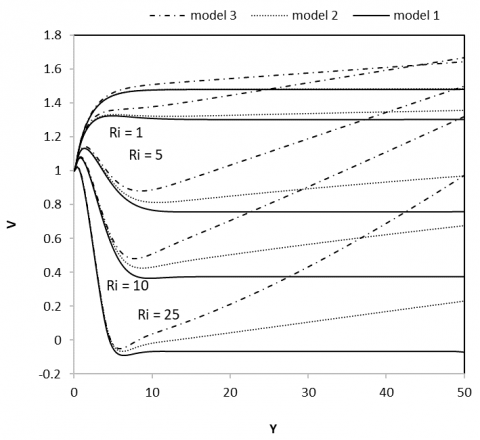Figure 8. Centerline axial velocity (dimensionless) in the channel using models 1, 2 and 3 for A=50, Re=150, Ri=0.1, 1, 5, 10, 25

It is observed from Figure 8 and 9, that centerline axial velocity and temperature difference (θW – θb) approaches towards constant value in model 1 which shows that the flow has become dynamically and thermally developed. But, model 2 and 3 predicts monotonically increasing axial velocity and monotonically increasing temperature difference (θW – θb) throughout the channel that shows dynamically and thermally developing flow throughout the channel, which thus represents the realistic condition in case of flow inside a vertical channel under constant wall heat flux condition . It is also observed that model 2 as compared to model 1 is closer to model 3 in predicting the axial velocity and temperature difference (θW – θb), therefore, model 2 shall predict better results as compared to model 1.

## 9.png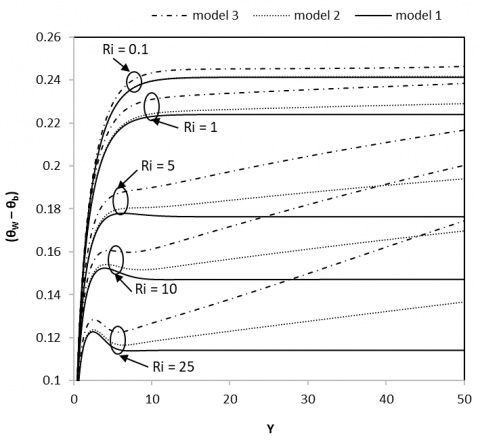Figure 9. θW – θb (dimensionless) distribution in the channel using models 1, 2 and 3 for A=50, Re=150, Ri=0.1, 1, 5, 10, 25

4.6 Streamline and isotherm profiles

The difference in flow appears amongst models 1, 2 and 3 as Ri increases. Therefore, streamlines and isotherms are presented here for Ri=25 at Re=150 and 300. Figure 10 shows streamlines (Figure 10a-c) and isotherms (Figure 10d-f) for Re=150, Ri=25 using models 1, 2 and 3. Model 1 (Figure 10a) shows parallel streamlines after inlet region and reversed flow takes place from top of the channel and reaches towards inlet region. Model 2 (Figure 10b) shows parallel streamlines near wall and converging streamlines in center region and also shows recirculatory cells in inlet region of the channel. Model 3 (Figure 10c) shows more converging stream-

lines in center region and shows recirculatory cells of smaller length as compared to that in model 2. Model 1 (Figure 10d) shows flatter isotherms in center region throughout the channel because of existence of reverse flow from channel top, while model 2 (Figure 10e) shows flatter isotherm from inlet to middle region of the channel and model 3 (Figure 10f) shows flatter isotherms in the inlet region of the channel only. The difference in isotherm profile of models 1, 2 and 3 is present due to the differences in reverse flow and recirculatory cells amongst models 1, 2 and 3 (Figure 10a-c). It is observed that with model 2 and 3, recirculatory cells appear with reverse flow. Formation of increased reverse was also evident in the work of Jha and Oni  for mixed convection flow in a vertical channel under asymmetric wall heating when density was allowed to vary non-linearly with temperature.

Figure 11 shows streamlines (Figure 11a-c) and isotherms (Figure 11d-f) for Re=300, Ri=25 using models 1, 2 and 3. Streamlines and isotherms for Re=300 appears relatively in the same manner for models 1, 2 and 3 as for Re=150. However, as Re increases to 300, reverse flow appears wider and elongated. Isotherm appears flatter and more dipped in center region of the channel as Re increases to 300.

## 11.pngFigure 10. Streamlines (a- model 1, b- model 2, c-model 3) and isotherms (d- model 1, e- model 2, f-model 3) in the channel at Re=150, Ri=25 for A=50

## 11.png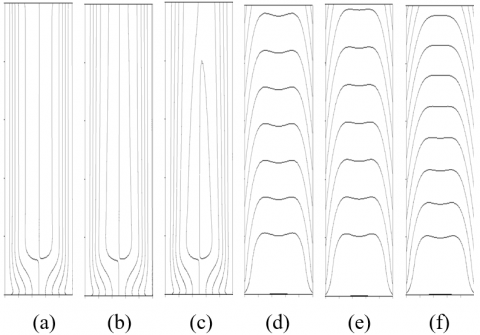Figure 11. Streamlines (a- model 1, b- model 2, c-model 3) and isotherms (d- model 1, e- model 2, f-model 3) in the channel at Re=300, Ri=25 for A=50

4.7 Regime of reverse flow

Regime of reverse flow can be described using Richardson number (Ri) and Peclet number (Pe) as described by Desrayaud and Lauriat  using ratio of Grashof and Reynolds number (Gr/Re) and Peclet number (Pe). Figure 12 shows Ri-Pe plot for finding the regime of reversed flow using models 1, 2 and 3. It is observed that as Pe decreases, Ri increases for reverses flow to start, which is observed in all models 1, 2 and 3. For a given Pe, reverse flow is started at lower value of Ri in model 1, while in model 3 reverse flow is started at higher value of Ri, and in model 2 reverse flow is started at Ri value which lies in between the Ri value of model 1 and 3. It is observed that Ri-Pe profile of model 2 as compared to model 1 is closer to that of model 3.

## 12.png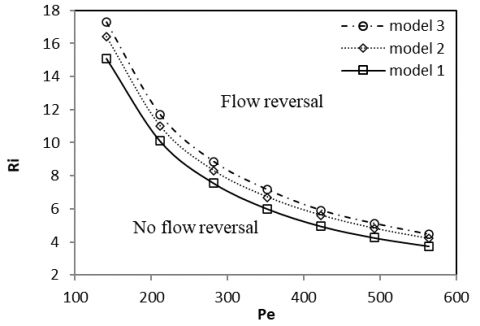Figure 12. Ri-Pe plot for regime of reverse flow using models 1, 2 and 3

Ri value for flow reversal to start for Pe value varied from 140 to 563 has been obtained for models 1, 2 and 3 are as follows,

$\text { Model 1: } R i \geq-0.0741+\frac{2140}{P e}$       (18)

$\text { Model 2: } R i \geq 0.1730+\frac{2286}{P e}$       (19)

$\text { Model 3: } R i \geq 0.0741+\frac{2411}{P e}$       (20)

5. Conclusions

Numerical investigation of laminar mixed convection flow in a vertical parallel plate channel under symmetric constant wall heat flux is performed by modelling the density using the equation of state without first-order approximation of density with respect to temperature. Here, model 3 (variable thermophysical properties) is taken as a reference model for finding the better model between model 1 (where $B=\operatorname{Ri} \theta$ ) and model 2 (where $\mathrm{B}=\rho^{*} \operatorname{Ri} \theta$ ) for the fluid which obeys ideal gas of equation. It is observed from velocity and temperature field, that results of model 2 as compared to model 1 is closer to the results of model 3 for various values of governing parameters, Re=150 and 300, Ri=0.1 to 25. Model 2 as compared to model 1 also successfully describes that in case of the channel flow with constant wall heat flux, velocity and temperature profile never become constant which correctly describes physics of the flow. In this study, appearance of recirculatory cells during reverse flow of model 2 and 3 are also observed in vertical channel flow with constant wall heat flux boundary condition, which was not reported earlier. Therefore, model 2 as compared to model 1 is relatively better in describing the mixed convection flow for the fluid which obeys the ideal gas equation of state.

Nomenclature
 A aspect ratio, h/w B buoyancy term Cfy local friction factor Cfavg average friction factor cp specific heat capacity of the fluid, J/kgK Fr Froude number, v∞/(gw)0.5 Gr Grashof number, βgΔTref w3/ ν2 g acceleration due to gravity, m/s2 h height of the channel, m kf thermal conductivity of the fluid, W/mK M number of grid points in X direction N number of grid points in Y direction Nuy local Nusselt number based on bulk temperature Nuy, local Nusselt number based on ambient temperature Nuavg average Nusselt number based on bulk temperature Nuav average Nusselt number based on ambient temperature p pressure, N/m2 P dimensionless pressure, p/ρv∞2 Pr Prandtl number, ν/α q heat flux, W/m2 k Thermal conductivity of the fluid, W/mK Re Reynolds number, v∞w/ν Ri Richardson number, Gr/Re2 T temperature at any location, K u horizontal velocity, m/s U dimensionless horizontal velocity v vertical velocity, m/s V dimensionless vertical velocity w channel width, m x,y distances in horizontal and vertical directions X,Y dimensionless horizontal and vertical distances, x/w, y/w respectively Greek symbols α thermal diffusivity of fluid, m2/s β volumetric expansion coefficient of the fluid, β = -(1/ρ)(∂ρ/∂T)p, K-1 ΔTref reference temperature difference, ΔTref = qw/kf, K µ dynamic viscosity of the fluid, kg/ms ν kinematic viscosity of fluid, m2/s ρ density of fluid, kg/m3 θ dimensionless temperature at any location in the computation domain, (T - T∞) / ΔTref Subscripts avg average b bulk c centreline f fluid w wall ∞ ambient ref reference avg average * dimensionless ratio of a variable with respect to inlet condition
References

 Aung, W., Worku, G. (1987). Mixed convection in ducts with asymmetric wall heat fluxes. Journal of Heat Transfer 109(4): 947-951. https://doi.org/10.1115/1.3248208

 Aung, W., Worku, G. (1986). Developing flow and flow reversal in a vertical channel with asymmetric wall temperatures. Journal Heat Transfer, 108(2): 299-304. https://doi.org/10.1115/1.3246919

 Habchi, S., Acharya, S. (1986). Laminar mixed convection in a symmetrically or asymmetrically heated vertical channel. Numerical Heat Transfer, Part A: Applications, 9(5): 605-618. https://doi.org/10.1080/10407788608913496

 Ingham, D.B., Keen, D.J., Heggs, P.J. (1988). Two dimensional combined convection in vertical parallel plate ducts, including situations of flow reversal. International Journal for Numerical Methods in Engineering, 26(7): 1645-1664. https://doi.org/10.1002/nme.1620260713

 Jeng, Y.N., Chen, J.L., Aung, W. (1992). On the Reynolds-number independence of mixed convection in a vertical channel subjected to asymmetric wall temperatures with and without flow reversal. International Journal of Heat and Fluid Flow, 13(4): 329-339. https://doi.org/10.1016/0142-727X(92)90003-R

 Cheng, C.H., Weng, C.J., Aung, W. (2000). Buoyancy-assisted flow reversal and convective heat transfer in entrance region of a vertical rectangular duct. International Journal of Heat and Fluid Flow, 21(4): 403-411. https://doi.org/10.1016/S0142-727X(00)00005-9

 Moukalled, F., Doughan, A., Acharya, S. (2000). Parametric study of mixed convection in channels with concave and convex surfaces. International Journal of Heat and Mass Transfer, 43(11): 1947-1963. https://doi.org/10.1016/S0017-9310(99)00269-0

 Premachandran, B., Balaji, C. (2006). A correlation for mixed convection heat transfer from converging, parallel and diverging channels with uniform volumetric heat generating plates. International Communications in Heat and Mass Transfer, 33(3): 350-356. https://doi.org/10.1016/j.icheatmasstransfer.2005.12.001

 Desrayaud, G., Lauriat, G. (2009). Flow reversal of laminar mixed convection in the entry region of symmetrically heated, vertical plate channels. International Journal of Thermal Sciences, 48(11): 2036-2045. https://doi.org/10.1016/j.ijthermalsci.2009.03.002

 Dritselis, C.D., Iatridis, A.J., Sarris, I.E., Vlachos, N.S. (2013). Buoyancy-assisted mixed convection in a vertical channel with spatially periodic wall temperature. International Journal of Thermal Sciences, 65: 28-38. https://doi.org/10.1016/j.ijthermalsci.2012.10.006

 Zghal, M., Galanis, N., Nguyen, C.T. (2001). Developing mixed convection with aiding buoyancy in vertical tubes: A numerical investigation of different flow regimes. International Journal of Thermal Sciences, 40(9): 816-824. https://doi.org/10.1016/S1290-0729(01)01268-6

 Lawrence, W.T., Chato, J.C. (1966). Heat-transfer effects on the developing laminar flow inside vertical tubes. Journal Heat Transfer, 88(2): 214-222. https://doi.org/10.1115/1.3691518

 Gray, D.D., Giorgini, A. (1976). The validity of the Boussinesq approximation for liquids and gases. International Journal of Heat and Mass Transfer, 19(5): 545-551. https://doi.org/10.1016/0017-9310(76)90168-X

 Fu, W.S., Chao, W.S., Peng, T.E., Li, C.G. (2016). Flow downward penetration of vertical parallel plates natural convection with an asymmetrically heated wall. International Communications in Heat and Mass Transfer, 74: 55-62. https://doi.org/10.1016/j.icheatmasstransfer.2016.03.006

 Talukdar, D., Li, C.G., Tsubokura, M. (2019). Investigation of compressible laminar natural-convection for a staggered and symmetric arrangement of discrete heat sources in an open-ended vertical channel. Numerical Heat Transfer, Part A: Applications, 76(3): 115-138. https://doi.org/10.1080/10407782.2019.1627833

 Greif, R. (1978). An experimental and theoretical study of heat transfer in vertical tube flows. Journal Heat Transfer, 100(1): 86-91. https://doi.org/10.1115/1.3450508

 Jha, B.K., Oni, M.O. (2019). Theory of fully developed mixed convection including flow reversal: A nonlinear Boussinesq approximation approach. Heat Transfer—Asian Research, 48(8): 3477-3488. https://doi.org/10.1002/htj.21550

 Rajeev, A., Mahanthesh, B. (2021). Multilayer flow and heat transport of nanoliquids with nonlinear Boussinesq approximation and viscous heating using differential transform method. Heat Transfer, 50(5): 4309-4327. https://doi.org/10.1002/htj.22076

 Patankar, S.V. (1980). Numerical Heat Transfer and Fluid Flow, Hemisphere Publ. Corp., New York, 58.

 Versteeg, H.K., Malalasekera, W. (2007). An Introduction to Computational Fluid Dynamics: The Finite Volume Method. Pearson Education.

 Tao, W.Q., Qu, Z.G., He, Y.L. (2004). A novel segregated algorithm for incompressible fluid flow and heat transfer problems—Clear (coupled and linked equations algorithm revised) part I: Mathematical formulation and solution procedure. Numerical Heat Transfer, Part B: Fundamentals, 45(1): 1-17. https://doi.org/10.1080/1040779049025485

 Elenbaas, W. (1942). Heat dissipation of parallel plates by free convection. Physica, 9(1): 1-28. https://doi.org/10.1016/S0031-8914(42)90053-3